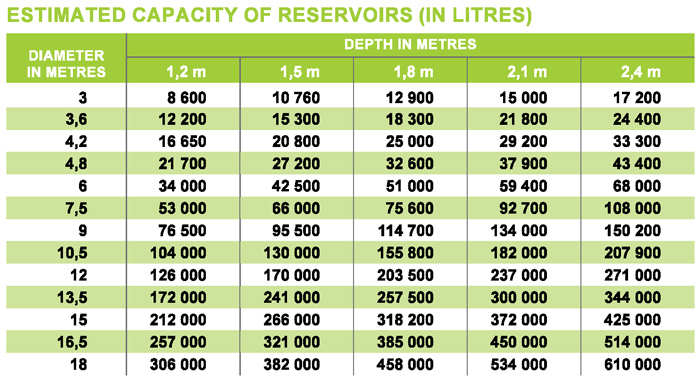# Measurement, Conversion Tables and Formulae

1046

September 2020 –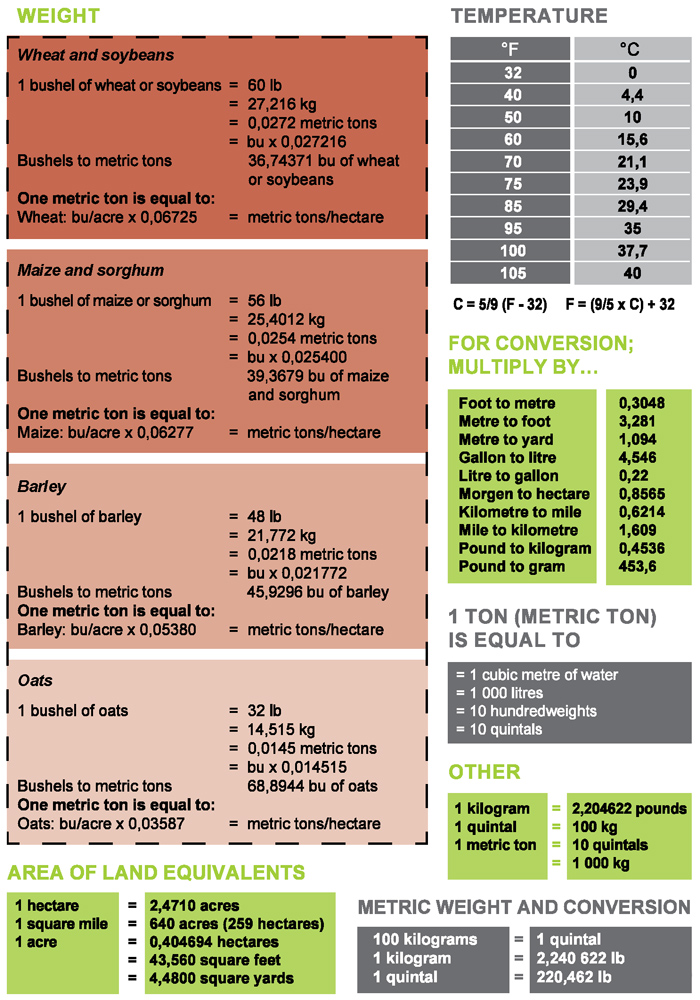Moisture content on delivery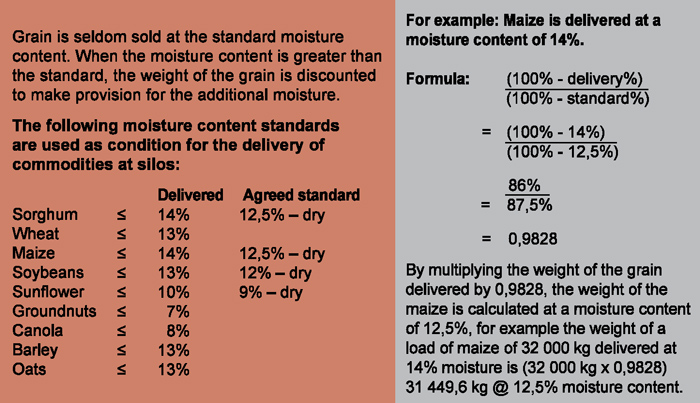CALCULATING WATER VOLUME

There are a few measurements that you need:

• Surface area
• Maximum depth

SURFACE AREA
The surface area of a dam is calculated by multiplying the length by the width.

Example: 30 m x 50 m = 1 500 m2

This will be the case for square or rectangular dams. Most dams start out as a square or rectangle, but over time appear to become rounded. If the dam is not exactly square, round the measurements off. Make sure to err on the conservative side.

Dams such as Turkey Nest dams (used to store water on a relatively flat terrain) can be any shape, and their dimensions may need to be approximated to calculate the surface area.

Gully dams (used to store water in a gully) can also be of varying shapes.

VOLUME
With the surface area depth calculated, the volume can then be determined:

Volume (m3) = surface area (m2) x maximum depth (m) x 0,4

(Where 0,4 accounts for the batter slope on the sides of the dam)

Example: 1 500 (m2) x 5 (m) x 0,4 = 3 000 m3

To calculate the capacity of your dam in mega litres (ML), divide the volume in m3 by 1 000 e.g. 3 000 m3 / 1 000 = 3 ML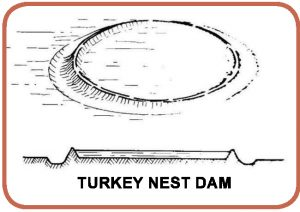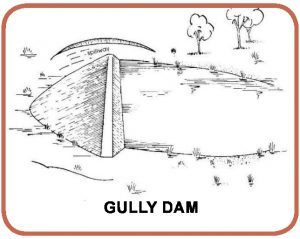Gully dams
Estimate the capacity of small gully storages using this formula:

Volume = (width x maximum depth x length) / 5 (where 5 is the correction factor)

Example: Water level height up bank = 2 m
Length = 20 m, width = 10 m
Volume of excavation = 100 m3
Volume = (2 x 20 x 10 + 100)/5 = 100 m3

Width and depth are measured at the embankment site and length is the distance water will back-up (you will need to add the volume of any excavations made below water level to give the total storage capacity). If the excavation volume is not known, substitute the depth of water at the deepest point as an approximation for the depth.

DEPTH
One way to determine dam depth is to row out into the dam and lower a weighted line over the side. When the line is vertical, measure the length of the line needed to reach the bottom. Alternatively, use a pole with distances marked on it. You will need to do this at a number of places across the dam to find the deepest point.

An alternative for smaller dams, or if no boat is available, use a fishing line with a sinker on the bottom with a float attached. The line is cast out repeatedly, with the float gradually adjusted until it’s not quite floating on the surface. The distance between the float and the sinker will be the depth at that point in the dam. Again, you will need to do this at a number of places across the dam.

Reference:
Rob Dimsey. December 2006. Vegetable-matters-of-fact, Number 43